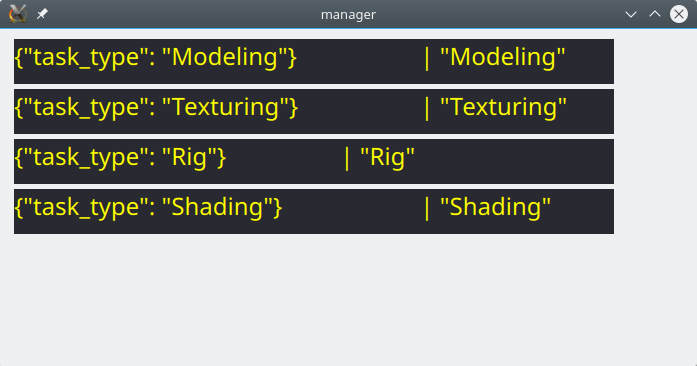# QSqlQueryModel with Open Cypher query issue

• Hi all!
I implemented simple model-view program which work with AgensGraph (fork of the PostgreSQL) base .
View part work on QML code. Model implemented on C++.
It is class inherited from QSqlQueryModel . Code was written by Qt Docs Example with one difference - query use OpenCypher instead SQL.
It's pretty well work with Qt 5.5.1 but not work with Qt 5.11.2

code for query:

`````` const char* ProjectBaseModel::SQL_SELECT =
"SET graph_path = prod; "
" match (n:task) return properties(n) AS n, n.task_type as t ;";
``````

Application connectedto the base but query not executed. It seems like empty query.
As you can see below it implemented in a refresh() method

``````void ProjectBaseModel::refresh()
{
this->setQuery(SQL_SELECT);
}
``````

The OpenCypher code is work in console connected with base and even in the Pgadmin.
Result of the query is:

``````n                           |  t
----------------------------+-------------
{"task_type": "Modeling"}   | "Modeling"
{"task_type": "Texturing"}  | "Texturing"
{"task_type": "Rig"}        | "Rig"
{"task_type": "Shading"}    | "Shading"
``````

I want to note also : the application work if I use SQL query to the tables from my base.
Have somebody same issues?

Thanks,
Alex

• Hi and welcome to devnet,

Did you check if you get any errors from the query and it's execution ?

• Hi SGaist.
My application don't generate any errors.
I had insert qDebug() calls for catch some states of some elements of the program
When I am compile it in the Qt5.5.1 application work and show my ListView with model data. I attached pic bellow.And application output is:

``````--Data Base Info after connection------------------------
db.tables() =  ("testTable", "world", "projects", "prod.ag_edge", "prod.ag_vertex", "prod.task", "prod.dependency", "pg_catalog.ag_graph", "pg_catalog.ag_label")
db.databaseName() =  "atmosphere_db"
db.connectionName() =  "qt_sql_default_connection"
db.driverName() =  "QPSQL"
--End Data Base Info -------------------------

--- Start roleNames() ---------
------------
roleName idx =  0 "n"
------------
roleName idx =  1 "t"
--- End roleNames() ---------
this->roleNames() =  QHash((258, "t")(257, "n"))
this->rowCount() =  4
QueryText =  SET graph_path = prod;  match (n:task) return properties(n) AS n, n.task_type as t ;
query.lastError() =  QSqlError("", "", "")
this->rowCount() =  4
--- Start roleNames() ---------
------------
roleName idx =  0 "n"
------------
roleName idx =  1 "t"
--- End roleNames() ---------
QModelIndex modelIndex =  QModelIndex(0,0,0x0,ProjectBaseModel(0x7fff3a984130))
QModelIndex modelIndex =  QModelIndex(0,1,0x0,ProjectBaseModel(0x7fff3a984130))
QModelIndex modelIndex =  QModelIndex(1,0,0x0,ProjectBaseModel(0x7fff3a984130))
QModelIndex modelIndex =  QModelIndex(1,1,0x0,ProjectBaseModel(0x7fff3a984130))
QModelIndex modelIndex =  QModelIndex(2,0,0x0,ProjectBaseModel(0x7fff3a984130))
QModelIndex modelIndex =  QModelIndex(2,1,0x0,ProjectBaseModel(0x7fff3a984130))
QModelIndex modelIndex =  QModelIndex(3,0,0x0,ProjectBaseModel(0x7fff3a984130))
QModelIndex modelIndex =  QModelIndex(3,1,0x0,ProjectBaseModel(0x7fff3a984130))
``````

When I compile the application in the Qt5.11.2 output is:

``````--Data Base Info after connection------------------------
db.tables() =  ("testTable", "world", "projects", "prod.ag_edge", "prod.ag_vertex", "prod.task", "prod.dependency", "pg_catalog.ag_graph", "pg_catalog.ag_label")
db.databaseName() =  "atmosphere_db"
db.connectionName() =  "qt_sql_default_connection"
db.driverName() =  "QPSQL"
--End Data Base Info -------------------------

--- Start roleNames() ---------
------------
roleName idx =  0 "n"
------------
roleName idx =  1 "t"
--- End roleNames() ---------
this->roleNames() =  QHash((257, "n")(258, "t"))
this->rowCount() =  0
QueryText =  SET graph_path = prod;  match (n:task) return properties(n) AS n, n.task_type as t ;
query.lastError() =  QSqlError("", "", "")
this->rowCount() =  0
``````

Alex

• Would it be possible for you to provide a minimal compilable example that creates a dummy database to reproduce this behaviour ?

• @SGaist Are you mean exapmle of OpenCypher commands for creating database or my Qt code for reading from base? I can share both.

• This is my OpenSypher code for creation the graph:

``````atmosphere_db=# CREATE GRAPH prod;
CREATE GRAPH
atmosphere_db=#
SET graph_path = prod;
CREATE VLABEL task;
CREATE ELABEL dependency;
CREATE (:task {task_type: 'Modeling'})-[:dependency {dep_type:'FS'}]->(:task {task_type: 'Texturing'});
CREATE (:task {task_type: 'Rig'})-[:dependency {dep_type:'FS'}]->(:task {task_type: 'Shading'});
``````

• @SGaist Are you mean exapmle of OpenCypher commands for creating database or my Qt code for reading from base? I can share both.

I meant a minimal application that would create the DB and do the access that are now failing.

• @SGaist Hi. But I created the base from console in Agens app (like psql). It was my first testing of Qt with Agens graph.
I wrote simplest code for catching the source of issue. But not found something yet. It work in the Qt5.5 and nor work in the Qt5.11.2

``````int main(int argc, char *argv[])
{
QCoreApplication a(argc, argv);

QSqlDatabase db = QSqlDatabase::addDatabase("QPSQL");
//QSqlDatabase db = QSqlDatabase::database();
db.setDatabaseName("atmosphere_db");
db.setUserName("atmosphere_user");
db.setHostName("localhost");
db.setPassword("atmosphere");
if (!db.open()) {
qDebug() << "Cannot open database:";
return 1;
}

qDebug() << "--Data Base Info after connection--------------";
qDebug() << "db.tables() = " << db.tables();
qDebug() << "db.databaseName() = " << db.databaseName();
qDebug() << "db.connectionName() = " << db.connectionName();
qDebug() << "db.driverName() = " << db.driverName();
qDebug() << "--End Data Base Info -------------------------" << "\n";

QSqlQuery q(db);
qDebug() << q.lastError();

q.exec("SET graph_path = prod; match (n:task) return properties(n) AS n, n.task_type as t ;");

qDebug() << q.lastError();

while (q.next()) {
qDebug() <<  q.value(0).toString() << "|" << q.value(1).toString() ;

}

return a.exec();
}
``````

• Finally I solved this issue. The queries pretty works if it do through separate "exec" calls. I wrote an example below

``````query = QSqlQuery(db);
query.exec("SET graph_path = some_graph; ");
query.exec("MATCH some OpenCypher match RETURN some Match variable;");
``````

• Sorry, I lost track on this one.

Glad you found out and thanks for sharing !

Since you have it working now, please mark the thread as solved using the "Topic Tools" button so that other forum users may know a solution has been found :)